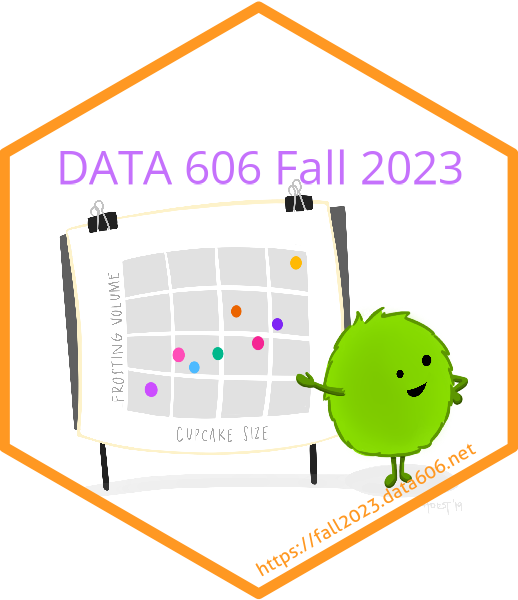# Chapter 3

## Probability

### Learning Outcomes

• Define trial, outcome, and sample space.
• Define and describe the law of large numbers.
• Distinguish disjoint (also called mutually exclusive) and independent events.
• Use Venn diagrams to represent events and their probabilities.
• Describe probability distributions.
• Distinguish between marginal and conditional probabilities.
• Use tree diagrams and/or Bayes Theorem to calculate conditional probabilities and probabilities of intersection of non-independent events.
• The expected value of a discrete random variable is computed by adding each outcome weighted by its probability.
$$E(X)=\mu=\sum_{i=1}^{k}{{x}_{i}P\left(X={x}_{i}\right)}$$
• The variance of a discrete random variable is computed by adding each squared deviation of an outcome from the expected value weighted by its probability. The standard deviation is the square root of the variance.
$$Var(X)={\sigma}^{2}=\sum_{i=1}^{k}{{\left({x}_{i}-\mu\right)}P\left(X={x}_{i}\right) }$$
• The average of a linear combination of discrete random variables is computed as the sum of their averages, weighted by the constant multipliers.
• The variance of a linear combination of independent discrete random variables is computed as the sum of their variances, weighted by the square of the constant multipliers.
• The distribution of a continuous random variable is described by the probability density function.
• The total area under the density curve is 1.
• Probabilities under the density curve can be calculated as the area under the curve.
• The probability of a continuous random variable being exactly equal to a value is 0, since there is no area under the curve at a given location.